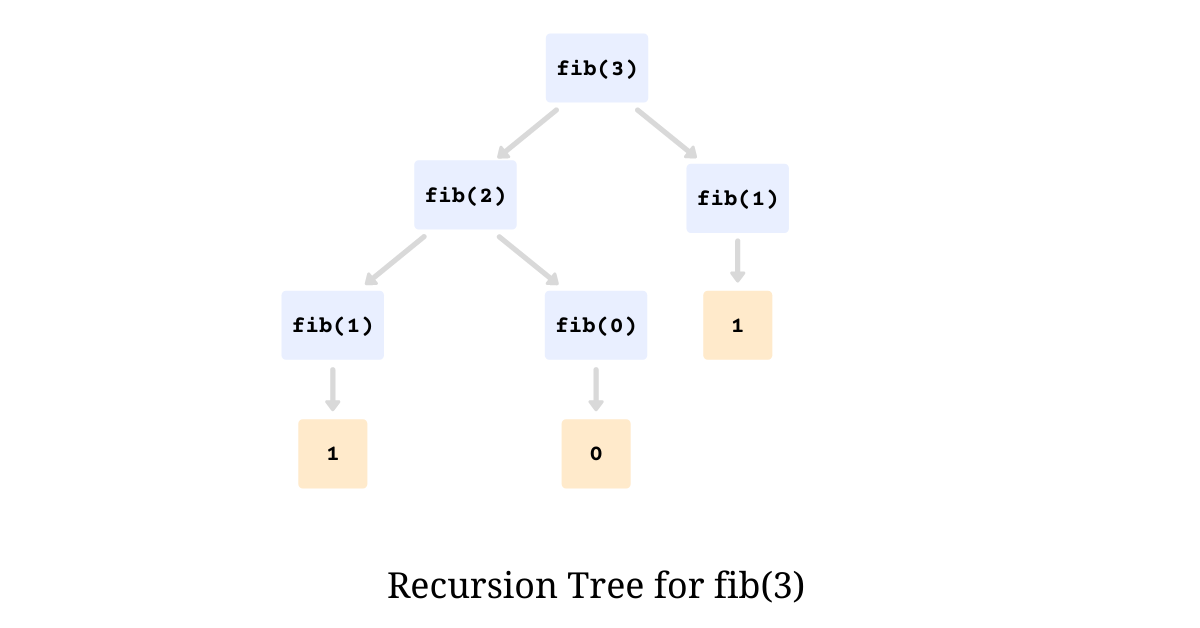Registrations for the November 2021 System Design Cohort are now open# A Simple Recursion Tree Visualizer for Python

Published on 13th Dec 2020

One programming paradigm, that is hardest to visualize, as almost every single programmer out there will agree on, is Recursion. We usually use pen and paper to visualize the flow and check how recursion is behaving. But what if, this could be done programmatically, and today we address this very problem and try to come up with a simple yet effective solution.

This essay is going to be a little different from the usuals; instead of taking a look into a research paper or an algorithm, we will implement a simple and easy recursion visualizer for Python.

# Recursion Tree

Recursion helps in solving a larger problem by breaking it into smaller similar ones. The classic implementation of recursion in the world of programming is when a function invokes itself using reduced parameters while having a base terminating condition.

``````def fib(n):
# base condition mimicking the first two numbers
# in the sequence
if n == 0: return 0
if n == 1: return 1

# every number is summation of the previous two
return fib(n - 1) + fib(n - 2)
``````

The most common problem that is solved using recursion is computing the `n`th Fibonacci Number. A trivial recursive Python function that spits out `n`th Fibonacci Number is as shown below

The most effective way of visualizing recursion is by drawing a recursion tree. It is very useful for visualizing what happens when a recurrence is iterated. The recursion tree for the above function `fib` for input `n = 3` is as illustrated below# Decorating to visualize

Instead of printing an actual tree-like recursion tree, we take some liberty and print a close-enough version of it running top-down. To keep track of recursive function calls we use Python Decorators that essentially wraps the function allowing us to invoke statements before and after the function call.

The decorator that wraps the recursive function and prints the recursion tree is as illustrated below.

``````def recviz(fn):
"""Decorator that pretty prints the recursion tree with
args, kwargs, and return values.
"""

# holds the current recursion level
recursion_level = 1

def wrapper(*args, **kwargs):

# we register a nonlocal recursion_level so that
# it binds with the recursion_level variable.
# in this case, it will bind to the one defined
# in recviz function.
nonlocal recursion_level

# Generate the pretty printed function string
fn_str = pretty_func(fn, args, kwargs)

# Generate the whitespaces as per the recursion level
whitespace = "   " * (recursion_level - 1)

# Pretty print the function with the whitespace
print(f"{whitespace} -> {fn_str}")

# increment the recursion level
recursion_level += 1

# Invoke the wrapped function and hold the return value
return_value = fn(*args, **kwargs)

# Post function evaluation we decrease the recursion
# level by 1
recursion_level -= 1

# Pretty print the return value
print(f"{whitespace} <- {repr(return_value)}")

# Return the return value of the wrapped function
return return_value

return wrapper
``````

We use `recursion_level` to keep track of the current recursion level using which we decide the indentation. The value of this variable is increased every time we are about the invoke the function while it is reduced post the execution. In order to pretty-print the invoked function, we have a helper method called `pretty_func` whose implementation can be found here.

When we decorate our previously defined `fib` function and invoke it with `n = 3` we get the following output.

`````` -> fib(3)
-> fib(2)
-> fib(1)
<- 1
-> fib(0)
<- 1
<- 2
-> fib(1)
<- 1
<- 3
``````

The above output renders how recurrence is evaluated and is pretty printed to make it more human-readable. The right arrow `->` defines a function invocation while the left arrow `<-` indicates the return value post invocation.

## Publishing it on PyPI

Everything mentioned above is published in a Python Package and hosted on PyPI at pypi/recviz. So in order to use this, simply install the package `recviz` like a usual Python package using `pip` and decorate the recursive function.

``````from recviz import recviz

@recviz
def fib(n):
# base condition mimicking the first two numbers
# in the sequence
if n == 0: return 0
if n == 1: return 1

# every number is summation of the previous two
return fib(n - 1) + fib(n - 2)

fib(3)
``````

# References

If my work adds value, consider supporting me## 2000+ Subscribers

If you like what you read subscribe you can always subscribe to my newsletter and get the post delivered straight to your inbox. I write essays on various engineering topics and share it through my weekly newsletter 👇

# Other articles that you might likeBuilding Finite State Machines with Python Coroutines

The most intuitive way of building and implementing Finite State Machines is by using Python Corouti...

19th AprInverse Document Frequency

TF-IDF is extensively used in search engines and in various document classification and clustering t...

6th MarConstant Folding in Python

Every programming language aims to be performant and Python is no exception. In this essay, we dive ...

10th JanWhy MongoDB's cursor.skip() is Slow?

MongoDB's cursor.skip() is very inefficient, why is that? Even though it is slow and inefficient, t...

4th Jun